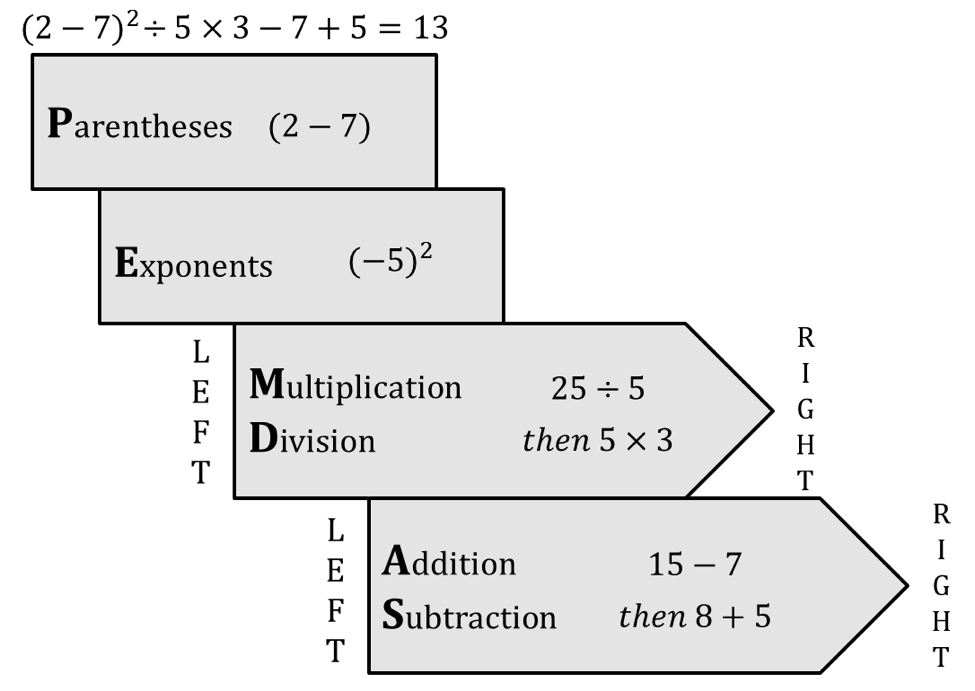Operations are the actions taken to solve math problems: addition, subtraction, multiplication, division, grouping (placing values in parentheses), and exponentiation (multiplying the same number to itself a certain number of times). Order of Operations was developed to make sure operations in a problem are completed in a particular order to achieve the correct result.

### The Order of Operations (PEMDAS)

1. Parentheses
2. Exponents
3. Multiplication
4. Division